## RD Sharma Class 8 Solutions Chapter 7 Factorizations Ex 7.5

These Solutions are part of RD Sharma Class 8 Solutions. Here we have given RD Sharma Class 8 Solutions Chapter 7 Factorizations Ex 7.5

Other Exercises

Factorize each of the following expressions :
Question 1.
16x2-25y2
Solution:
16x2 – 25y2 = (4x)2 – (5y)2    {∵ a2 – b2 = (a + b) (a – b)}
= (4x + 5y) (4x – 5y)

Question 2.
27x2 12y2
Solution:
27x2 – 12y2 = 3 (9x2 – 4y2)  {∵ a2 -b2 = (a + b) (a – b)}
= 3 [(3x)2 – (2y)2]
= 3 (3x + 2y) (3x – 2y)

Question 3.
144a– 289b2
Solution:
144a2 – 289b2 = (12a)2 – (17b)2    { ∵ a2b2 = (a + b) (a – b}
= (12a+ 17b) (12a- 17b)

Question 4.
12m2 – 27
Solution:
12m2 – 27 = 3 (4m2 – 9)
= 3 {(2m)2-(3)2}   {∵ a2b2 = (a + b) (a – b)}
= 3 (2m + 3) (2m – 3)

Question 5.
125x2 – 45y2
Solution:
125x2 – 45y2 = 5 (25x2 – 9y2)
= 5 {(5x-)2 – (3y)2}    {∵ a2 – b2 = (a + b) (ab}
= 5 (5x +
3y) (5x – 3y)

Question 6.
144a2 – 169b2
Solution:
144a2 – 169b2 = (12a)2 – (13b)2    {∵ a2 -b2 = (a + b) (a – b)}
= (12a + 13b) (12a-13b)

Question 7.
(2a – b)2 – 16c2
Solution:
(2a – b)2 – 16c2 = (2a – b)2 – (4c)2   {∵ a2 – b2 = (a + b) (a – b)}
= (2a – b + 4c) (2a – b – 4c)

Question 8.
(x + 2y)2 – 4 (2x -y)2
Solution:
(x + 2y)2 – 4 (2x – y)2
= (x + 2y)2 – {2 (2x –y)}2
=
(x + 2y)2 – (4x – 2y)2        {∵ a2– b2 = (a + b) (a – b)}
=
(a + 2y + 4x – 2y) (x + 2y – 4x + 2y)
= 5x (-3x + 4y)

Question 9.
3a5 – 48a3
Solution:
3a5 – 48a3 = 3a3 (a2– 16)
= 3a3 {(a)2 – (4)2}        {∵ a2 – b2 = (a + b) (a – b)}
= 3a3 (a + 4) (a – 4)

Question 10.
a4 – 16b4
Solution:
a4 – 16b4 = (a2)2 – (4b2)2
= (a2 + 4b2) (a2 – 4b2)
= (a2 + 4b2) {(a)2 – (2b)2 }   { ∵ a2 – b2 = (a + b) (a – b)}
= (a2 + 4b2) (a + 2b) (a – 2b)

Question 11.
x8 – 1
Solution:
x8 – 1 = (x4)2 – (1)2
= (x4 + 1) (x4 – 1)
= (x4+ 1) I (x2)2 – (1)2}             { a2 – b2 = (a + b) (a – b)}
= (x4 + 1) (x2 + 1) (x2 – 1)
= (x4 + 1) (x2 + 1) {(x)2 – (1)2}
= (x4+ 1)(x2 + 1)(x+ 1)(x- 1)
= (x-1)(x+ 1) (x2 + 1) (x4 + 1)

Question 12.
64 – (a + 1)2
Solution:
64 – (a + 1)2 = (8)2 – (a + 1)2    {∵ a2 – b2 = (a + b) (a – b)}
= (8 + a + 1) (8 – a – 1)
= (9 + a) (7 – a)

Question 13.
36l2 – (m + n)2
Solution:
36l2 – (m + n)2 = (6l)2 – (m + n)2        {∵  a2 – b2 = (a + b) (a – b)}
= (6l + m + n) (6l – m – n)

Question 14.
25x4y4 – 1
Solution:
25x4y4 – 1 = (5x4y4)2 – (1)2         { ∵  a2 – b2 = (a + b) (a – b)}
= (5x4y4  + 1) (5x2y2  – 1)

Question 15.Solution: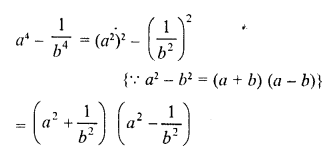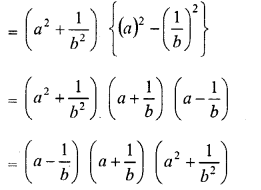Question 16.
x3 – 144x
Solution:
x3 – 144x = x (x2 – 144)
= x {(x)2 – (12)2}       { a2 – b2 = (a + b) (a – b)}
=  x (x + 12) (x – 12)

Question 17.
(x – 4y)2 – 625
Solution:
(x – 4y)2 – 625
= (x – 4y)2 – (25)2     {∵ a2 – b2 = (a + b) (a – b)}
= (x – 4y + 25) (x -4y – 25)

Question 18.
9 (a – b)2 – 100 (x -y)2
Solution:
9(a-b)2– 100(x-y)2
= {3(a-b)}2-{10(x-y)}2      { a2 – b2 = (a + b) (a – b)}
= (3a – 3b)2 – (10x – 10y)2
= (3a – 3b + 10x – 10y) (3a – 3b – 10x + 10y)

Question 19.
(3 + 2a)2 – 25a2
Solution:
(3 + 2a)2 – 25a2
= (3 + 2a)2 – (5a)2      ( a2 – b2 = (a + b) (a – b)}
= (3 + 2a + 5a) (3 + 2a – 5a)
= (3 + 7a) (3 – 3a)
= (3 + 7a) 3 (1 – a)
= 3(1-a) (3 +7a)

Question 20.
(x + y)2 – (a – b)2
Solution: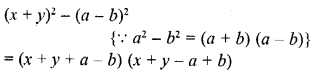Question 21.Solution: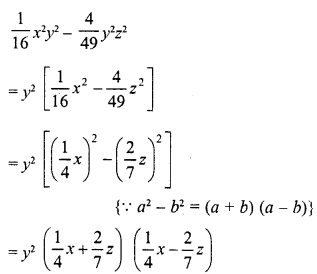Question 22.
75a3b2 – 108ab4
Solution:
75a3b2 – 108ab4
= 3ab2 (25a2 – 36b2)
= 3ab2 {(5a)2 – (6b)2}         { a2 – b2 = (a + b) (a – b)}
= 3ab2 (5a + 6b) (5a – 6b)

Question 23.
x5– 16x3
Solution:
x5 – 16x3 = x3 (x2 – 16)
= x3 {(x)2 – (4)2} { a2 – b2 = (a + b) (a – b)}
= x3 (x + 4) (x – 4)

Question 24.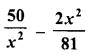Solution:Question 25.
256x5 – 81x
Solution:
256x5– 81x = x(256x4– 81)
= x {(16x2)2 – (9)2}      {∵ a2 – b2 = {a + b) (a – b)}
= x (16x2 + 9) (16x2 – 9)
= x (16x2 + 9) {(4x)2 – (3)2}
= x (16x2 + 9) (4x + 3) (4x-3)

Question 26.
a4 – (2b + c)4
Solution:
a4 – (2b + c)4
= (a2)2 – [(2b + c)2]2    { a2 – b2 = (a + b) (a – b)}
= {a2 + (2b + c)2} {a2 – (2b + c)2}
= {a2 + (2b + c)2} {(a)2 – (2b + c)2}
= {a2 + (2b + c)2} (a + 2b + c) (a -2b- c)

Question 27.
(3x + 4y)4 – x4
Solution:
(3x + 4y)4 – x4 – [(3x + 4y)2]2 – (x2)2
= [(3x + 4y)2 + x2] [(3x + 4y)2 – x2]       {∵  a2 – b2 = (a + b) (a – b)
= [(3x + 4y)2 + x2] [(3x + 4y + x) (3x + 4y – x)]
=   [(3x + 4y)2 + x2] (4x + 4y) (2x + 4y)
= [(3x + 4y)2 + x2] 4 (x + y) 2 (x + 2y)
= 8 (x + y) (x + 2y) [(3x + 4y)2 + x2]

Question 28.
p2q2 – p4q4
Solution:
p2q2– p4q4 =p2q2 (1 -p2q2)
=p2q2 [(1)2 – (pq)2]   { a2 – b2 = (a + b) (a – b)
= p2q2 (1 +pq) (1 -pq)

Question 29.
3x3y – 243xy3
Solution:
3x3y – 243xy3
= 3xy (x2 – 81y2)
= 3xy [(x)2 – (9y)2]
= 3xy (x + 9y) (x – 9y)

Question 30.
a4b4 – 16c4
Solution:
a4b4 – 16c4 = (a2b2)2 – (4c2)2
= (a2b2 + 4c2) (a2b2 – 4c2)
= (a2b2 + 4c2) [(ab)2 – (2c)2]      { a2 – b2 = (a + b) (a – b)
= (a2b2 + 4c2) (ab + 2c) (ab – 2c)

Question 31.
x4-625
Solution:
x4 – 625 = (x2)2 – (25)2   { a2 – b2 – (a + b) (a – b)
= (x2 + 25) (x2 – 25)
= (x2 + 25) [(x)2 – (5)2]
= (x2 + 25) (x + 5) (x – 5)

Question 32.
x4-1
Solution:
x4 – 1 = (x2)2 – (1)2 = (x2 + 1) (x2 – 1)
= (x2 + 1) [(x)2 – (1)2]
= (x2 + 1) (x + 1) (x – 1)

Question 33.
49 (a – b)2 -25 (a + b)2
Solution:
49 (a – by -25 (a + b)2
= [7 (a – b)]2 [5 (a + b)]2
= (7a – 7b)2 – (5a + 5b)2  { a2 – b2 = (a + b) (a – b)
= (7a -7b + 5a + 5b) (7a – 7b -5a- 5b)
=(12a – 2b)(2a – 12b)
= 2 (6a – b) 2 (a – 6b)
= 4 (6 a- b) (a – 6b)

Question 34.
x – y – x2 + y
Solution:
x-y-x2 + y2 = (x-y)-(x2-y2) {∵ a2 – b2 = (a + b) (a – b)
= {x-y)-(x + y)(x-y)
= (x-y)(1 – x – y)

Question 35.
16 (2x – 1)2 – 25y2
Solution:
16 (2x – 1)2 – 25y2
= [4 (2x – 1)]2 – (5y)2
= (8x – 4)2 – (5y)2
= (8x – 4 + 5y) (8x -4-5y)
= (8x + 5y – 4) (8x – 5y – 4)

Question 36.
4 (xy + 1)2 – 9 (x – 1)2
Solution:
4 (xy + 1)2 – 9 (x – 1)2
=
[2 (xy + 1)]2 – [3 (x – 1)]2
= (2xy + 2)2 – (3x – 3){∵ a2 – b2 = (a + b) (a – b)
= (2xy + 2 + 3x – 3) (2xy + 2 – 3x + 3)
= (2xy + 3x – 1) (2xy – 3x + 5)

Question 37.
(2x + 1)2 – 9x4
Solution:
(2x + 1)2 – 9x4 = (2x + 1)2 – (3x2)2    { a2 – b2 = (a + b) (a – b)
= (2x + 1 + 3x2) (2x + 1 – 3x2)
= (3x2 + 2x + 1) (-3x + 2x + 1)

Question 38.
x4 – (2y- 3z)2
Solution:
x4 – (2y – 3z)2 = (x2)2 – (2y – 3z)2
= (x2 + 2y- 3z) (x2 – 2y + 3z)

Question 39.
a2-b2 +a-b
Solution:
a2 – b2 + a – b
= (a + b) {a – b) + 1 (a – b)
= (a – b) (a + b + 1)

Question 40.
16a4 – b4
Solution:
16a4 – b4
= (4a2)2 – (b2)2            {   a2 – b2 = (a + b) (a – b)
= (4a2 + b2) (4a2 – b2)
= (4a2 + b2) {(2a)2 – (b)2}
= (4a2 + b2) (2a + b) (2a – b)

Question 41.
a4 – 16 (b – c)4
Solution:
a4 – 16 (b- c)4 = (a2)2 – [4 (b – c)2]{   a2 – b2 = (a + b) (a – b)
= [a2 + 4 (b – c)2] [a2 – 4 (b – c)2]
= [a2 + 4 (b – c)2] [(a)2 – [2 (b – c)]2]
= [a2 + 4 (b – c)2] [(a)2 – (2b – 2c)2]
= [a2 + 4 (b – c)2] (a + 2b – 2c) (a – 2b + 2c)

Question 42.
2a5 – 32a
Solution:
2a5 – 32a = 2a (a4 – 16)
= 2a [(a2)2 – (4)2]  {∵  a2 – b2 = (a + b) (a – b)
= 2a (a2 + 4) (a2 – 4)]
= 2a (a2 + 4) [(a)2 – (2)2]
= 2a (a2 + 4) (a + 2) (a – 2)

Question 43.
a4b4 – 81c4
Solution:
a4b4 – 81c4 = (a2b2)2 – (9c2)2
= (a2b2 + 9c2) (a2b2 – 9c2
{∵ a2 – b2 = (a + b) (a – b)
= (a2b2 + 9c2) {(ab)2 – (3c)2}
= (a2b2 + 9c2) (ab + 3c) (ab – 3c)

Question 44.
xy9-yx9
Solution:
xy9yx9 = xy (y8 – x8)
= xy [(y4)2 – (x4)2{∵  a2 – b2 = (a + b) (a – b)}
= xy(y4 + x4)(y4-x4)
= xy (y4 + x4) {(y2)2 – (x2)2}
= xy (y4 + x4) (y2 + x2) (y2 – x2)
= xy (y4 + x4) (y2 + x2) (y + x) (y – x)

Question 45.
x3 -x
Solution:
x3-x = x(x2 1)
= x [(x)2 – (1)2] = x (x + 1) (x – 1)

Question 46.
18a2x2 – 32
Solution:
18a2x2 – 32
= 2 [9a2x2 – 16]
= 2 [(3ax)2 – (4)2]   { a2 – b2 = (a + b) (a – b)
= 2 (3ax + 4) (3ax – 4)

Hope given RD Sharma Class 8 Solutions Chapter 7 Factorizations Ex 7.5 are helpful to complete your math homework.

If you have any doubts, please comment below. Learn Insta try to provide online math tutoring for you.